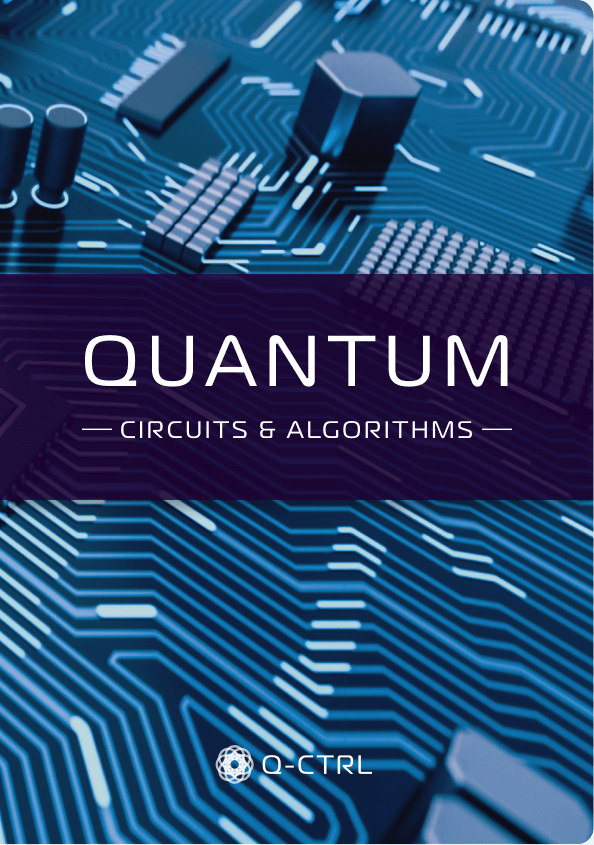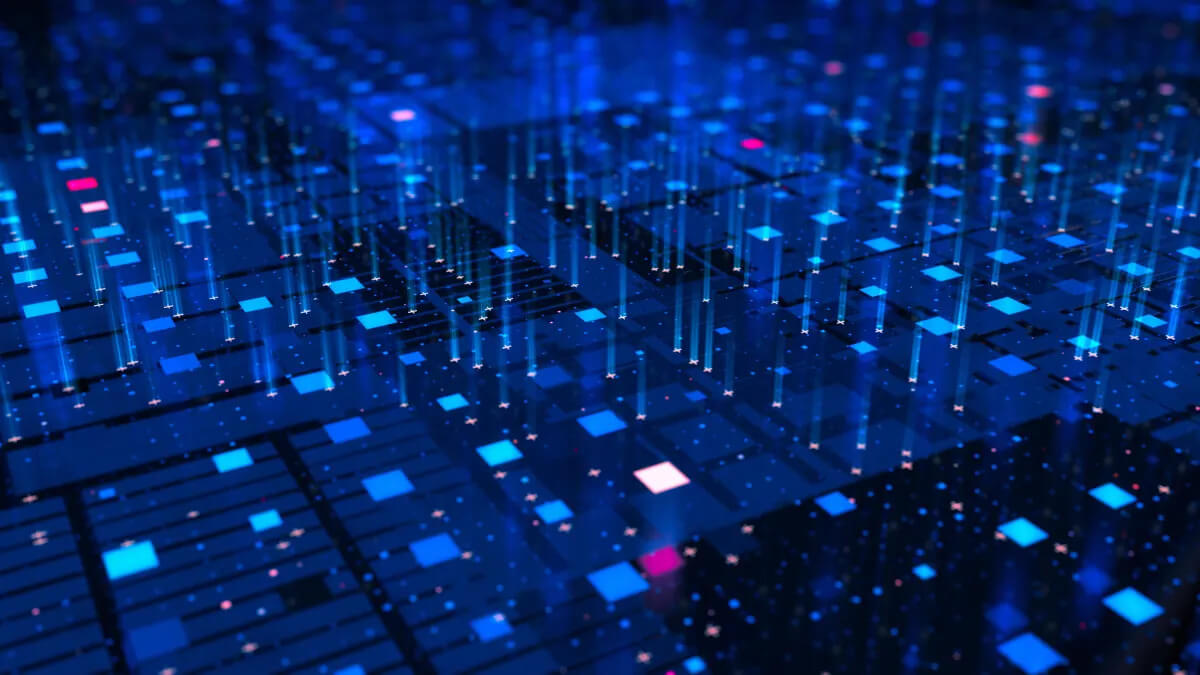Beginner

# What are quantum algorithms?

Learn the fundamentals of how to build quantum algorithms for quantum computing - starting with quantum circuits and logic through to how they're measured.#### REPRESENTING ALGORITHMS IN A QUANTUM COMPUTER

In this article we’ll introduce you to how we build and represent algorithms in a quantum computer.

#### QUANTUM CIRCUITS

In the field of quantum computing we use a simple visual tool called a quantum circuit to illustrate how an algorithm or program is executed. These circuits are a bit different than the circuits you might see in electronics which show connections in space. In quantum circuits we show connections between qubits in space as well as operations performed in time. If you’ve ever played a musical instrument, you can think about a quantum circuit like a musical score that plays an algorithm as you read along.

The first critical elements are the horizontal rails which each represent individual qubits initialized in a starting state. Multiple qubits are represented by multiple rails. The horizontal axis then represents time. We can then add different quantum logic operations in order to build up a program. As time flows from left to right, the state of the set of qubits changes because of the operations.

#### QUANTUM LOGIC OPERATIONS

Now let’s look at the next critical elements - the quantum logic operations. Each of these is represented by a small symbol with a letter or short phrase inside. Some act on individual qubits, like bit flips (X), phase flips (Z), and Hadamard gates (H). Others are drawn as connecting two qubits together - common examples are the SWAP and CNOT gates. The CNOT gate is a very special form of conditional logic similar to the conventional XOR gate. These two-qubit gates are essential for actually building up the complex quantum state which is used to perform a computation inside the quantum computer. Due to its action it’s also known as an “entangling gate” which (under the right circumstances) generates entanglement between qubits.

#### MEASUREMENT

The final element is measurement. In quantum computers we generally only perform measurements at the end of a computation, because once we perform a measurement on a qubit, we destroy quantum superpositions. The result of any measurement on an individual qubit will be a 1 or a 0.

Consider a simple example. Let’s start with two qubits initialized in the state $|0 \rangle$, each represented as a rail. Now let’s perform a Hadamard gate on the first qubit. This transforms qubit one from a basis state to an equal superposition of the two basis states of the qubit. On the Bloch sphere that means it evolves from a pole to the equator. If you then apply a CNOT gate between the two qubits while the first is in a superposition, the result is a fundamentally different object. Instead of two separate qubits we now have an entangled pair in something called a Bell State.

There’s just no way to describe that classically as two separate qubits; the state now exists not as individual qubits but rather a mathematical description of the “correlations” between them. These correlations describe how the outcome of measurements on one qubit would be linked to measurements on the other. With this example, measuring 0 or 1 on one qubit would ALWAYS result in the same measurement outcome on the other. That’s because they aren’t really independent anymore!

That’s a pretty simple example that does something really interesting but not necessarily really useful. But if you add together enough qubits and enough operations in just the right way, you can actually perform something quite impactful...like Shor’s algorithm.

Building a useful algorithm requires us to manipulate the enormous superposition states of all qubits in such a way that even when we destroy everything at the end by measuring our qubits we still get a useful outcome. Shor’s algorithm is extremely clever because it figures out how to do this successfully. That’s great! But Shor’s algorithm is extremely complex to implement. Performing any useful factoring will require thousands of qubits each capable of performing perhaps trillions of operations as the algorithm runs.

Why aren’t we there yet? The answer is noise and error which cause quantum computers to fail.

## Foundations

Take the next step on your journey with short articles to help you understand how quantum computing and sensing will transform the world